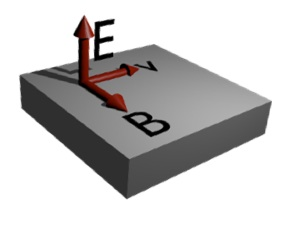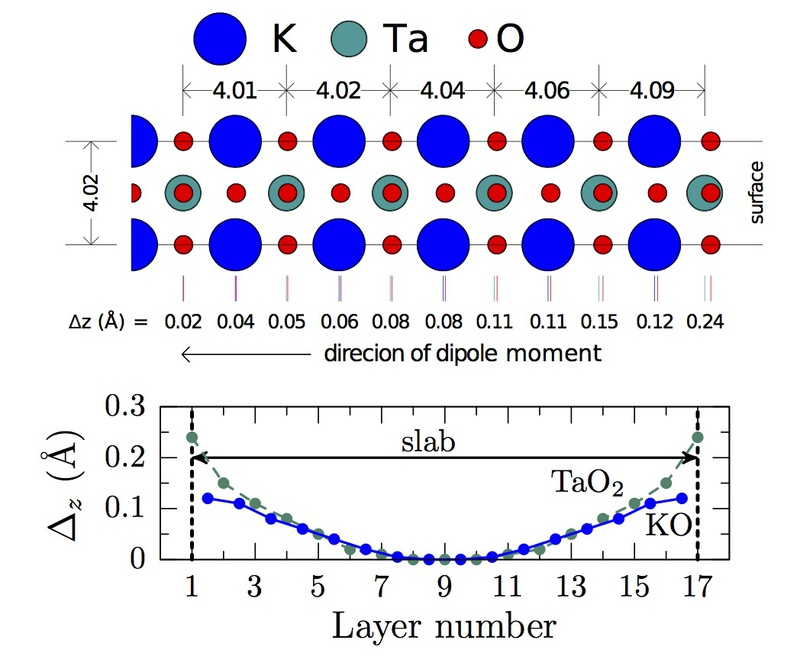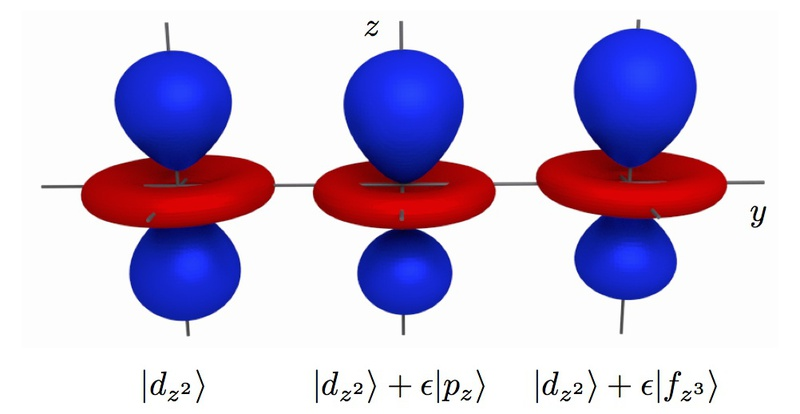# Rashba effectThe Rashba effect, or Rashba-Dresselhaus effect, is a momentum-dependent splitting of spin bands in two-dimensional condensed matter systems. Consider a particle with charge and spin moving with a velocity v through an electric field E. It feels a magnetic field B given by,$B = -\frac{v\times E}{c^2} = -\frac{p\times E}{m_cc^2}$

The spin of the particle will couple to this field through the Hamiltonian,$H_{SO} = -\mu_B\sigma\cdot (\frac{p\times E}{2m_cc^2})$

Which can be rewritten in the Rashba form:$H_R = \alpha_R(\hat{z}\times k)\cdot \sigma$Fig 1: Plots showing the 2D electron gas (2DEG) density (top) and the local potential (bottom) for slab of KTaO3 in the unrelaxed (left) and relaxed (right) structures obtained from density functional theory based calculations. The TaO2 layers are numbered in x axis and the surface layer is marked as 1. As the bottom panel shows, structural relaxation substantially weakens the surface potential.Fig 2: Relaxed structure of the KTaO3 slab in the first six layers near the surface, beyond which there was no significant distortion from the unrelaxed structure. These results are for no applied electric field. The lower panel shows the change of the cation-anion distance Δz in various layers due to relaxation, with layer numbers 1 and 17 being the two surface Ta layers.Fig 7: Contour plot of 3dz2 orbital showing the effect of the electric-field induced mixing with the pz and fz3 orbitals with ε = 0.3. The colors differentiate the signs of the wave function.Fig 10: Rashba spin splitting through second-order perturbation theory. The bands shown in the left are spin degen- erate. The dz2↑ band interacts with dxz↑ through electric field (2iγ1kx) while the dz2↓ band interacts dxz↑ through spin-orbit coupling (√3λ/2). Together, they cause splitting of the up and down bands as shown in the right. The curvature of dxz, dyz bands are taken to be negative for clarity.

27. Theoretical study of the cubic Rashba effect at the SrTiO3 (001) surfaces,
K. V. Shanavas
Phys. Rev. B 93, 045108 (2015) |
18. Electric Field Tuning of the Rashba Effect in the Polar Perovskite Structures
K. V. Shanavas, and S. Satpathy
Phys. Rev. Lett. 112, 086802 (2014) | |
• #### Categories:

Physics